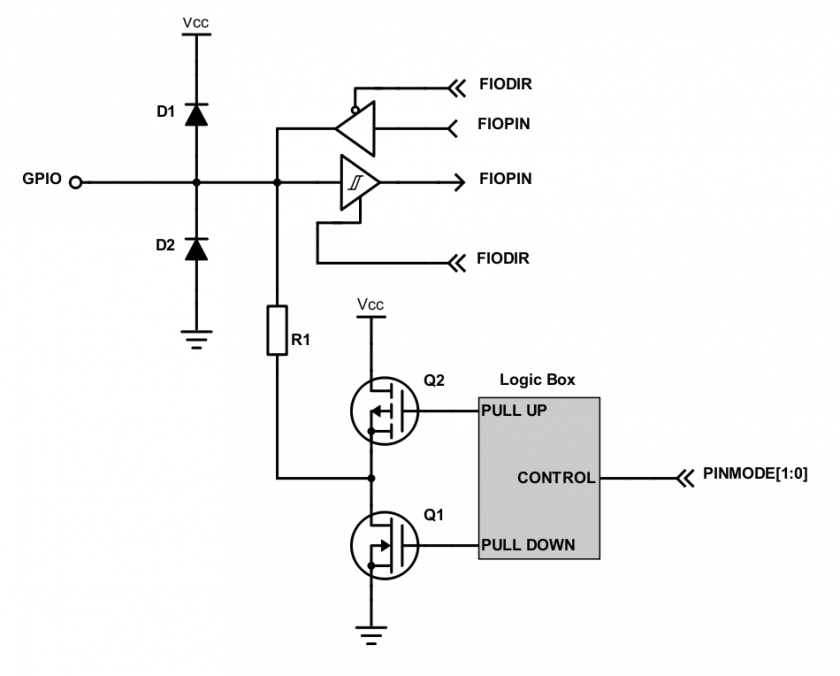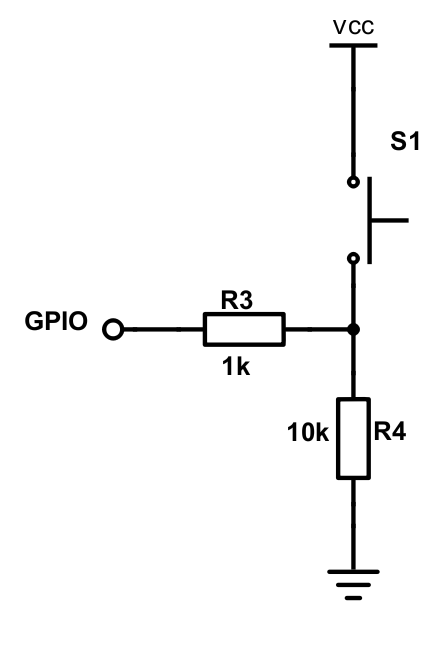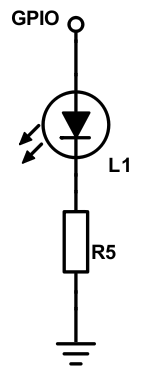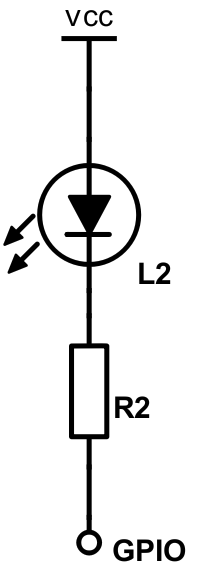# Lesson GPIO

Bit-masking is a technique to selectively modify individual bits without affecting other bits.

#### Bit SET

To set a bit, we need to use the OR operator. This is just like an OR logical gate you should've learned in the Digital design course. To set a bit, you would OR a memory with a bit number, and the bit number with which you will OR will end up getting set.

``````// Assume we want to set Bit#7 of a register called: REG
REG = REG | 0x80;

// Let's set bit#31:
REG = REG | 0x80000000;

// Let's show you the easier way:
// (1 << 31) means 1 gets shifted left 31 times to produce 0x80000000
REG = REG | (1 << 31);

// Simplify further:
REG |= (1 << 31);

// Set Bit#21 and Bit# 23:
REG |= (1 << 21) | (1 << 23);``````

#### Bit CLEAR

To reset or clear a bit, the logic is similar, but instead of ORing a bit, we will AND a bit. Remember that AND gate clears a bit if you AND it with 0 so we need to use a tilde (~) to come up with the correct logic:

``````// Assume we want to reset Bit#7 of a register called: REG
REG = REG &   0x7F;
REG = REG & ~(0x80); // Same thing as above, but using ~ is easier

// Let's reset bit#31:
REG = REG & ~(0x80000000);

// Let's show you the easier way:
REG = REG & ~(1 << 31);

// Simplify further:
REG &= ~(1 << 31);

// Reset Bit#21 and Bit# 23:
REG &= ~( (1 << 21) | (1 << 23) );``````

#### Bit TOGGLE

``````// Using XOR operator to toggle 5th bit
REG ^= (1 << 5);

// Invert bit3, and bit 5
REG ^= ((1 << 3) | (1 << 5));``````

#### Bit CHECK

Suppose you want to check bit 7 of a register is set:

``````if(REG & (1 << 7))
{
DoAThing();
}

// Loop while bit#7 is a 0
while( ! (REG & (1 << 7)) ) {
;
}``````

Now let's work through another example in which we want to wait until bit#9 is 0

``````// As long as bit#9 is non zero (as long as bit9 is set)
while((REG & (1 << 9)) != 0) {
;
}

// As long as bit#9 is set
while(REG & (1 << 9)) {
;
}
``````

### GPIO Example of NXP CPU

In this example, we will work with an imaginary circuit of a switch and an LED. For a given port, the following registers will apply:

• GPIO selection: PINSEL register (not covered by this example)
• GPIO direction: DIR (direction) register

Each bit of DIR1 corresponds to each external direction pin of PORT1. So, bit0 of DIR1 controls the direction of physical pin P1.0 and bit31 of DIR2 controls physical pin P2.31. Similarly, each bit of PIN controls the output high/low of physical ports P1 and P2. PIN not only allows you to set an output pin, but it allows you to read input values as sensed on the physical pins.

Suppose a switch is connected to GPIO Port P1.14 and an LED is connected to Port P1.15. Note that if a bit is set of DIR register, the pin is OUTPUT otherwise the pin is INPUT. So... 1=OUTPUT, 0=INPUT

``````// Set P1.14 as INPUT for the switch:
LPC_GPIO1->DIR &= ~(1 << 14);

// Set P1.15 as OUTPUT for the LED:
LPC_GPIO1->DIR |=  (1 << 15);

// Read value of the switch:
if(LPC_GPIO1->PIN & (1 << 14)) {
// Light up the LED:
LPC_GPIO1->PIN |= (1 << 15);
} else {
// Else turn off the LED:
LPC_GPIO1->PIN &= ~(1 << 15);
}``````

LPC also has dedicated registers to set or reset an IOPIN with hardware AND and OR logic:

``````if (LPC_GPIO1->PIN & (1 << 14)) {
LPC_GPIO1->SET = (1 << 15); // No need for |=
}
else {
// Else turn off the LED:
LPC_GPIO1->CLR = (1 << 15); // No need for &=
}``````

### Brainstorming

• How many ways to test an integer named value is a power of two by using a bit manipulation?
``````(value | (value + 1)) == value
(value & (value + 1)) == value
(value & (value - 1)) == 0
(value | (value + 1)) == 0
(value >> 1) == (value/2)
((value >> 1) << 1) == value ``````
• What does this function do?
``````boolean foo(int x, int y) {
return ((x & (1 << y)) != 0);
}``````

# GPIO

#### Objective

To be able to General Purpose Input Output (GPIO), to generate digital output signals, and to read input signals. Digital outputs can be used as control signals to other hardware, to transmit information, to signal another computer/controller, to activate a switch or, with sufficient current, to turn on or off LEDs, or to make a buzzer sound.

Below will be a discussion on using GPIO to drive an LED.

Although the interface may seem simple, you do need to consider hardware design and know some of the fundamentals of electricity. There are a couple of goals for us:

• No hardware damage if faulty firmware is written
• The circuit should prevent an excess amount of current to avoid processor damage.

#### Required Background

You should know the following:

• Wire-wrapping or use of a breadboard
• Fundamentals of electricity, such as Ohm's law (V = IR) and how diodes work.
##### GPIOFigure 1. Internal Design of a GPIO

GPIO stands for "General Purpose Input Output". Each pin can at least be used as an output or input. In an output  configuration, the pin voltage is either 0v or 3.3v. In input mode, we can read whether the voltage is 0v or 3.3v.

You can locate a GPIO that you wish to use for a switch or an LED by first starting with the schematic of the board. The schematic will show which pins are "available" because some of the microcontroller pins may be used internally by your development board. After you locate a free pin, such as P2.0, then you can look-up the microcontroller user manual to locate the memory that you can manipulate.

##### Hardware Registers Coding

The hardware registers map to physical pins. If we want to attach our switch and the LED to our microcontroller's PORT0, then reference the relevant registers and their functionality.

Note that in the LPC17xx, the registers had the words `FIO` preceding the `LPC_GPIO` data structure members. In the LPC40xx, the word `FIO` has been dropped. `FIO` was a bit historic and it stood for "Fast Input Output", but in the LPC40xx, this historic term was deprecated.

 LPC40xx Port0 Registers LPC_GPIO0->DIR The direction of the port pins, 1 = output LPC_GPIO0->PIN Read: Sensed inputs of the port pins, 1 = HIGHWrite: Control voltage level of the pin, 1 = 3.3v LPC_GPIO0->SET Write only: Any bits written 1 are OR'd with PIN LPC_GPIO0->CLR Write only: Any bits written 1 are AND'd with PIN
##### Switch

We will interface our switch to PORT0.2, or port zero's 3rd pin (counting from 0).

Note that the "inline" resistor is used such that if your GPIO is misconfigured as an OUTPUT pin, hardware damage will not occur from badly written software.Figure 2. Button Switch Circuit Schematic

``````/* Make direction of PORT0.2 as input */
LPC_GPIO0->DIR &= ~(1 << 2);

/* Now, simply read the 32-bit PIN register, which corresponds to
* 32 physical pins of PORT0.  We use AND logic to test if JUST the
* pin number 2 is set
*/
if (LPC_GPIO0->PIN & (1 << 2)) {
// Switch is logical HIGH
} else {
// Switch is logical LOW
}``````
##### LED

We will interface our LED to PORT0.3, or port zero's 4th pin (counting from 0).

Given below are two configurations of an LED. Usually, the "sink" current is higher than "source", hence the active-low configuration is used more often.Figure 3. Active High LED circuit schematicFigure 4. Active low LED circuit schematic

``````const uint32_t led3 = (1U << 3);

/* Make direction of PORT0.3 as OUTPUT */
LPC_GPIO0->DIR |= led3;

/* Setting bit 3 to 1 of IOPIN will turn ON LED
* and resetting to 0 will turn OFF LED.
*/
LPC_GPIO0->PIN |= led3;

/* Faster, better way to set bit 3 (no OR logic needed) */
LPC_GPIO0->SET = led3;

/* Likewise, reset to 0 */
LPC_GPIO0->CLR = led3;``````

# Lab: GPIO

##### Objective
• Manipulate microcontroller's registers in order to access and control physical pins
• Use implemented driver to sense input signals and control LEDs
• Use FreeRTOS binary semaphore to signal between tasks

In this portion of the lab, you will design a basic task structure, and directly manipulate the microcontroller register to blink an on-board LED. You will not need to implement a full GPIO driver for this part. Instead, directly manipulate registers from `LPC40xx.h`

`````` void led_task(void *pvParameters) {
// Choose one of the onboard LEDS by looking into schematics and write code for the below
0) Set the IOCON MUX function(if required) select pins to 000

1) Set the DIR register bit for the LED port pin

while (true) {
2) Set PIN register bit to 0 to turn ON LED (led may be active low)

3) Set PIN register bit to 1 to turn OFF LED
}
}

int main(void) {

return 0;
}``````

##### Part 1: GPIO Driver

Use the following template to implement a GPIO driver composed of:

• Source file

Implement ALL of the following methods. All methods must work as expected as described in the comments above their method name. Note that you shall not use or reference to the existing `gpio.h` or `gpio.c` and instead, you should build your own `gpio_lab.h` and `gpio_lab.c` as shown below.

``````// file gpio_lab.h
#pragma once

#include <stdint.h>
#include <stdbool.h>

// include this file at gpio_lab.c file
// #include "lpc40xx.h"

// NOTE: The IOCON is not part of this driver

/// Should alter the hardware registers to set the pin as input
void gpio0__set_as_input(uint8_t pin_num);

/// Should alter the hardware registers to set the pin as output
void gpio0__set_as_output(uint8_t pin_num);

/// Should alter the hardware registers to set the pin as high
void gpio0__set_high(uint8_t pin_num);

/// Should alter the hardware registers to set the pin as low
void gpio0__set_low(uint8_t pin_num);

/**
* Should alter the hardware registers to set the pin as low
*
* @param {bool} high - true => set pin high, false => set pin low
*/
void gpio0__set(uint8_t pin_num, bool high);

/**
* Should return the state of the pin (input or output, doesn't matter)
*
* @return {bool} level of pin high => true, low => false
*/
bool gpio0__get_level(uint8_t pin_num);``````

Extra Credit
Design your driver to be able to handle multiple ports (port 1 and port 2) within a single gpio driver file
Do this only after you have completed all of the lab

##### Part 2. Use GPIO driver to blink two LEDs in two tasks

This portion of the lab will help you understand the `task_parameter` that can be passed into tasks when they start to run. You will better understand that each task has its own context, and its own copies of variables even though we will use the same function for two tasks.

``````typedef struct {
/* First get gpio0 driver to work only, and if you finish it
* you can do the extra credit to also make it work for other Ports
*/
// uint8_t port;

uint8_t pin;
} port_pin_s;

// Type-cast the paramter that was passed from xTaskCreate()

while(true) {
gpio0__set_high(led->pin);

gpio0__set_low(led->pin);
}
}

int main(void) {
// TODO:
// Pass each task its own parameter:
// This is static such that these variables will be allocated in RAM and not go out of scope
static port_pin_s led0 = {0};
static port_pin_s led1 = {1};

return 0;
}``````

##### Part 3: LED and Switch
• Interface the switch and LED task with a Binary Semaphore
• Deprecated requirements:
• For this final portion of the lab, you will interface an external LED and an external switch
• Do not use the on-board LEDs for the final demonstration of the lab

• Hint: You can make it work with on-board LED and a switch before you go to the external LED and an external switch
##### Requirements:
• Do not use any pre-existing code or library available in your sample project (such as `gpio.h`)
• You should use memory mapped peripherals that you can access through `LPC40xx.h`
``````#include "FreeRTOS.h"
#include "semphr.h"

static SemaphoreHandle_t switch_press_indication;

while (true) {
// Note: There is no vTaskDelay() here, but we use sleep mechanism while waiting for the binary semaphore (signal)
if (xSemaphoreTake(switch_press_indication, 1000)) {
} else {
puts("Timeout: No switch press indication for 1000ms");
}
}
}

while (true) {
// TODO: If switch pressed, set the binary semaphore
if (gpio0__get_level(switch->pin)) {
xSemaphoreGive(switch_press_indication);
}

// Task should always sleep otherwise they will use 100% CPU
// This task sleep also helps avoid spurious semaphore give during switch debeounce
}
}

int main(void) {
switch_press_indication = xSemaphoreCreateBinary();

// Hint: Use on-board LEDs first to get this logic to work
//       After that, you can simply switch these parameters to off-board LED and a switch
static port_pin_s switch = {...};
static port_pin_s led = {...};

Upload only relevant `.c` files into canvas. A good example is: `main.c`, `lab_gpio_0.c`. See Canvas for rubric and grade breakdown.